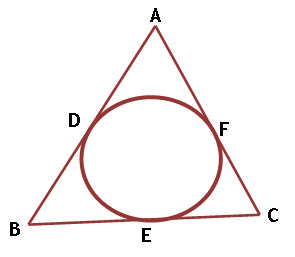Maths / Circles / Length of Tangents to a Circle

QUESTION

 In fig. if AB = AC, prove that BE = EC.EXPLANATION
Explain TypeExplanation Content
Text

Solution    As lengths of tangents from an external point to a circle are equal.

AD = AF            [ lengths of the tangents from point A ]

BD = BE            [ lengths of the tangents from point B ]

CE = CF            [ lengths of the tangents from point C ]

AD + BD + CE = AF + BE + CF

( AD + BD ) + CE = ( AF + CF ) + BE

AB + CE = AC + BE

AB + CE = AB + BE                       [   AC = AB ]

CE = BE                   [ cancel AB from both the sides ]

TestimonialsStudent Feedback on Methodology - Prisha Gupta C/o ABHYAS Academy
7th
This academy have a planned schedule and lessons are been taught in such a way that we learn many things to make a base for the next class.